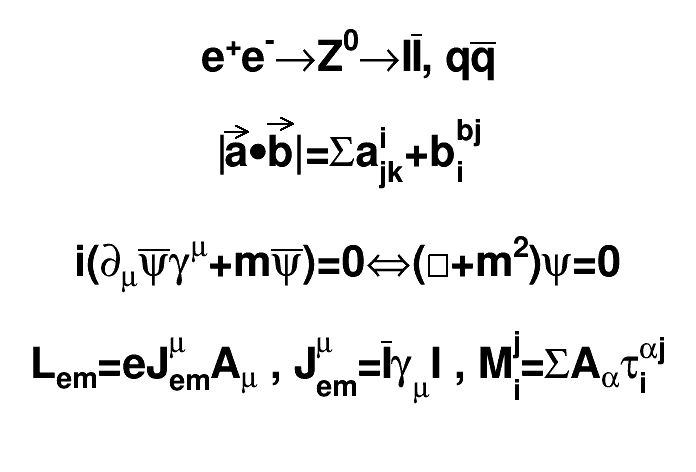# latex2¶

This macro draws 4 Latex-style formula in a canvas and prints the canvas as a Postscript file.

Author: Rene Brun
This notebook tutorial was automatically generated with ROOTBOOK-izer from the macro found in the ROOT repository on Monday, August 15, 2022 at 09:36 AM.

In :
TCanvas *c1 = new TCanvas("c1");
TLatex l;
l.SetTextAlign(23);
l.SetTextSize(0.1);
l.DrawLatex(0.5,0.95,"e^{+}e^{-}#rightarrowZ^{0}#rightarrowI#bar{I}, q#bar{q}");
l.DrawLatex(0.5,0.75,"|#vec{a}#bullet#vec{b}|=#Sigmaa^{i}_{jk}+b^{bj}_{i}");
l.DrawLatex(0.5,0.5,"i(#partial_{#mu}#bar{#psi}#gamma^{#mu}+m#bar{#psi})=0\
#Leftrightarrow(#Box+m^{2})#psi=0");
l.DrawLatex(0.5,0.3,"L_{em}=eJ^{#mu}_{em}A_{#mu} , J^{#mu}_{em}=#bar{I}\
#gamma_{#mu}I , M^{j}_{i}=#SigmaA_{#alpha}#tau^{#alphaj}_{i}");
c1->Print("latex2.ps");

Info in <TCanvas::Print>: ps file latex2.ps has been created


Draw all canvases

In :
gROOT->GetListOfCanvases()->Draw()